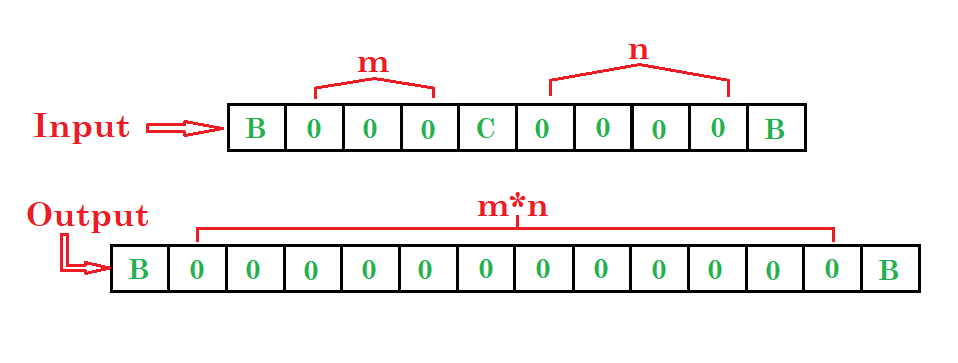# Turing machine for multiplication

• Difficulty Level : Medium
• Last Updated : 11 Jun, 2018

Prerequisite – Turing Machine
Problem: Draw a turing machine which multiply two numbers.

Example:

Attention reader! Don’t stop learning now.  Practice GATE exam well before the actual exam with the subject-wise and overall quizzes available in GATE Test Series Course.

Learn all GATE CS concepts with Free Live Classes on our youtube channel.Steps:

• Step-1. First ignore 0’s, C and go to right & then if B found convert it into C and go to left.

• Step-2. Then ignore 0’s and go left & then convert C into C and go right.

• Step-3. Then convert all X into X and go right if 0 found convert it into X and go to left otherwise if C found convert it into B and go to right and stop the machine.

• Step-4. If then X found convert it into X and go left then C into C and left then Y into Y and left.

• Step-5. Then if B found convert it into B and right then if Y into 0 and right or if C into C and right and go to step 3 and repeat the process otherwise if 0 found after 4th step then convert it into Y and right then Y into Y and right then C into C and right then 0 into 0 or X into X and right then C into C and right then 0 into 0 and right then B into 0 and left then 0 into 0 and left then C into C and left then 0 into 0 or X into X and left then C into C and left.

• Step-6. Then repeat the 5th step.Here, q0 shows the initial state and q1, q2, ….., q10, q11are the transition states and q12shows the final state.
And X, Y, 0, C are the variables used for multiplication and R, L shows right and left.

My Personal Notes arrow_drop_up